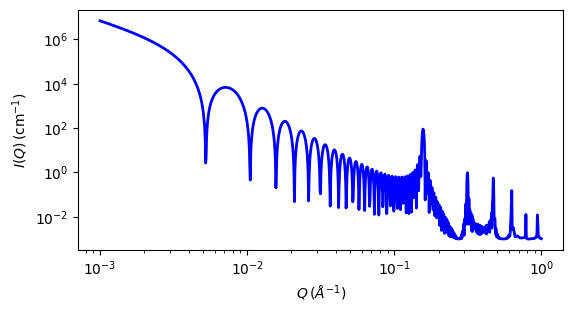# lamellar_hg_stack_caille

Parameter

Description

Units

Default value

scale

Scale factor or Volume fraction

None

1

background

Source background

cm-1

0.001

length_tail

Tail thickness

10

2

Nlayers

Number of layers

None

30

d_spacing

lamellar d-spacing of Caille S(Q)

40

Caille_parameter

Caille parameter

None

0.001

sld

Tail scattering length density

10-6-2

0.4

10-6-2

2

sld_solvent

Solvent scattering length density

10-6-2

6

The returned value is scaled to units of cm-1 sr-1, absolute scale.

This model provides the scattering intensity, $$I(q) = P(q)S(q)$$, for a lamellar phase where a random distribution in solution are assumed. Here a Caille $$S(q)$$ is used for the lamellar stacks.

The scattering intensity $$I(q)$$ is

$I(q) = 2 \pi \frac{P(q)S(q)}{q^2\delta }$

The form factor $$P(q)$$ is

$P(q) = \frac{4}{q^2}\big\{ \Delta\rho_H \left[\sin[q(\delta_H + \delta_T)] - \sin(q\delta_T)\right] + \Delta\rho_T\sin(q\delta_T)\big\}^2$

and the structure factor $$S(q)$$ is

$S(q) = 1 + 2 \sum_1^{N-1}\left(1-\frac{n}{N}\right) \cos(qdn)\exp\left(-\frac{2q^2d^2\alpha(n)}{2}\right)$

where

\begin{align*} \alpha(n) &= \frac{\eta_{cp}}{4\pi^2} \left(\ln(\pi n)+\gamma_E\right) && \\ \gamma_E &= 0.5772156649 && \text{Euler's constant} \\ \eta_{cp} &= \frac{q_o^2k_B T}{8\pi\sqrt{K\overline{B}}} && \text{Caille constant} \end{align*}

$$\delta_T$$ is the tail length (or length_tail), $$\delta_H$$ is the head thickness (or length_head), $$\Delta\rho_H$$ is SLD(headgroup) - SLD(solvent), and $$\Delta\rho_T$$ is SLD(tail) - SLD(headgroup). Here $$d$$ is (repeat) spacing, $$K$$ is smectic bending elasticity, $$B$$ is compression modulus, and $$N$$ is the number of lamellar plates (Nlayers).

NB: When the Caille parameter is greater than approximately 0.8 to 1.0, the assumptions of the model are incorrect. And due to a complication of the model function, users are responsible for making sure that all the assumptions are handled accurately (see the original reference below for more details).

Non-integer numbers of stacks are calculated as a linear combination of results for the next lower and higher values.

Be aware that the computations may be very slow.

The 2D scattering intensity is calculated in the same way as 1D, where the $$q$$ vector is defined as

$q = \sqrt{q_x^2 + q_y^2}$Fig. 48 1D plot corresponding to the default parameters of the model.

Source

References

1. F Nallet, R Laversanne, and D Roux, J. Phys. II France, 3, (1993) 487-502

2. J Berghausen, J Zipfel, P Lindner, W Richtering, J. Phys. Chem. B, 105, (2001) 11081-11088

Authorship and Verification

• Author: